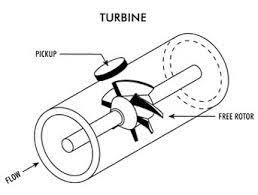# Turbine mass flowmeters## Impeller-Turbine Mass Flow Meters:

The impeller, turbine type mass flow meter uses two rotating elements in the fluid stream, an impeller and a turbine. Both elements contain channels through which the fluid flows.

The impeller is driven at a constant speed by a synchronous motor through a magnetic coupling and imparts an angular velocity to the fluid as it flows through the meter.

The turbine located downstream of the impeller eliminates all the angular momentum of the fluid and, therefore, receives a torque proportional to the angular momentum.

This turbine is constrained by a spring that deflects through an angle that is proportional to the torque exerted on it by the fluid, which gives a measure of mass flow.

## Twin-Turbine Mass Flow Meter:

In this instrument two turbines are mounted on a common shaft.

As shown in the above figure, a twin-turbine mass flow meter in which two turbines are connected with a calibration torsion member. A reluctance type pick up is mounted over each turbine and a strong magnet is located in each turbine within the twinturbine assembly.

Each turbine is designed with a different blade angle; therefore, there is a tendency for the turbines to rotate at different angular speeds.

However, since the movement of the turbines is restricted by the coupling torque, the entire assembly rotates in unison at an average speed, and an angular phase shift between the two turbines develops. This angle is a direct function of the angular momentum of the fluid

The angular momentum is a function of mass flow. In the double turbine assembly, the turbines are not restricted by a spring, but the torsion member that holds them together is twisted. Therefore, the angle developed between the two turbines is a direct function of the torsion or torsion exerted by the system.

Scroll to Top